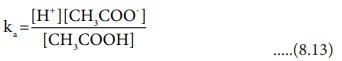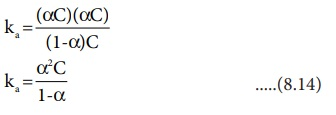Home | | Chemistry 12th Std | Ionisation of weak acids

# Ionisation of weak acids

OstwaldŌĆÖs dilution law relates the dissociation constant of the weak acid (Ka ) with its degree of dissociation (╬▒) and the concentration (c).

Ionisation of weak acids

We have already learnt that weak acids are partially dissociated in water and there is an equilibrium between the undissociated acid and its dissociated ions.

Consider the ionisation of a weak monobasic acid HA in water.

HA + H2O Ōåö H3O+ + A-

Applying law of chemical equilibrium, the equilibrium constant Kc is given by the expressionThe square brackets, as usual, represent the concentrations of the respective species in moles per litre.

In dilute solutions, water is present in large excess and hence, its concentration may be taken as constant say K. Further H3O+ indicates that hydrogen ion is hydrated, for simplicity it may be replaced by H+ . The above equation may then be written as,The product of the two constants KC and K gives another constant. Let it be KaThe constant Ka is called dissociation constant of the acid. Like other equilibrium constants, Ka also varies only with temperature.

Similarly, for a weak base, the dissociation constant can be written as below.## OstwaldŌĆÖs dilution law

OstwaldŌĆÖs dilution law relates the dissociation constant of the weak acid (Ka ) with its degree of dissociation (╬▒) and the concentration (c). Degree of dissociation (╬▒) is the fraction of the total number of moles of a substance that dissociates at equilibrium.

╬▒= Number of moles dissociated / total number of molesWe shall derive an expression for ostwald's law by considering a weak acid, i.e. acetic acid (CH3COOH). The dissociation of acetic acid can be represented as

CH3COOH Ōåö H+ + CH3COO-

The dissociation constant of acetic acid is,Substituting the equilibrium concentration in equation (8.13)We know that weak acid dissociates only to a very small extent. Compared to one, ╬▒ is so small and hence in the denominator (1 - ╬▒) 1. The above expression (8.14) now becomes,

Ka =╬▒2CLet us consider an acid with Ka value 4 ├Ś 10-4 and calculate the degree of dissociation of that acid at two different concentration 1 ├Ś 10-2M and 1 ├Ś 10-4M using the above expression (8.15)

For 1 ├Ś 10-2M ,i.e, When the dilution increases by 100 times, (Concentration decreases from 1 ├Ś 10-2M to 1 ├Ś 10-4M ), the dissociation increases by 10 times.

Thus, we can conclude that, when dilution increases, the degree of dissociation of weak electrolyte also increases. This statement is known as OstwaldŌĆÖs dilution Law.

The concentration of H + (H 3O+ ) can be calculated using the Ka value as below.

[ H+]= ╬▒C    (Refer table) .....(8.16)

Equilibrium molar concentration of [H+ ] is equal to ╬▒C### Example 8.4

A solution of 0.10M of a weak electrolyte is found to be dissociated to the extent of 1.20% at 25oC . Find the dissociation constant of the acid.

Given that ╬▒=1.20%=1.20/100

= 1.2 ├Ś 10-2

Ka = ╬▒2c

= (1.2 ├Ś 10-2 )2 (0.1) = 1.44 ├Ś10-4 ├Ś10-1

= 1.44 ├Ś 10-5

### Example 8.5

Calculate the pH of 0.1M CH3COOH solution. Dissociation constant of acetic acid is 1.8 ├Ś10-5 .

pH=-log[H+ ]

For weak acids,

[H+ ]= ŌłÜ [Ka ├Ś C]

= ŌłÜ (1.8 ├Ś10-5 ├Ś 0.1)

=1.34 ├Ś10-3M      pH=-log (1.34 ├Ś10-3)

= 3 - log1.34

= 3 - 0.1271

= 2.8729 Ōēł2.87

Evaluate yourself ŌĆō 7

Kb for NH 4 OH is 1.8 ├Ś 10-5 . Calculate the percentage of ionisation of 0.06M ammonium hydroxide solution.

╬▒ = ŌłÜ(Kb / C)  = 1.8 ├Ś10-5 /  6 ├Ś 10-2

= ŌłÜ 3├Ś10-4

= 1.732 ├Ś 10-2

= 1.732 / 100 = 1.732%

Tags : OstwaldŌĆÖs dilution law | Ionic Equilibrium | Chemistry , 12th Chemistry : UNIT 8 : Ionic Equilibrium
Study Material, Lecturing Notes, Assignment, Reference, Wiki description explanation, brief detail
12th Chemistry : UNIT 8 : Ionic Equilibrium : Ionisation of weak acids | OstwaldŌĆÖs dilution law | Ionic Equilibrium | Chemistry